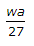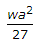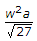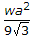# Civil Engineering - Theory of Structures - Discussion

### Discussion :: Theory of Structures - Section 1 (Q.No.19)

19.

A simply supported beam carries varying load from zero at one end and w at the other end. If the length of the beam is a, the maximum bending moment will be

 [A].[B].[C].[D].Explanation:

No answer description available for this question.

 Ferd said: (Apr 27, 2016) It should be :(2wa^2)/(9 * square root of 3).

 Prem Khandeep said: (Feb 13, 2017) According to me, It is wl^2÷9 √3EI.

 Muzzamil said: (Jul 25, 2017) It is 2wl/9*√ 3.

 Lokesh Sahu said: (Aug 5, 2017) Let reaction loads are RA & RB @ point A & B. RA+RB=wl/2. To calculating we found RA= wl/6 & RB= -wl/3, let take a section X-X @ a distance of x from A. UVL will be @ X-X = wx/l, & load on triangle ACX = wx2 /2l, & find the SF @ X-X, Fx= RA - load on triangle ACX, Fx=( wl/6) - (wx2/2l) -------> (1) Fx at A will be. X=0 Fx = wl/6 Fx at B will be. X=l Fx=-wl/3. Moment will be max, where Fx=0, So, x= l/(3)1/2. Now find the moment at X-X. Mx = (RA*x) " (load on triangle ACX * x/3)load of the triangle will be act ta 1/3rd distance from A. Mx= (wlx/6) " (wx3/6l) Moment at x= l/(3)1/2 Mx= (wl*( l/(3)1/2)/6) - (w*( l/(3)1/2)3/6l) Mx= wl2/9(3)1/2.

 Nami said: (Apr 2, 2018) Thanks @Lokesh. Really good explanation.

 Ankit said: (Feb 22, 2019) Well said, Thanks, @Lokesh.

 Sandeep Kshetri said: (Mar 31, 2019) How the reaction at support b is negative? it would be positive.

 Vaibhav Tatawar said: (Nov 19, 2020) Thanks for explaining @Llokesh Sahu.